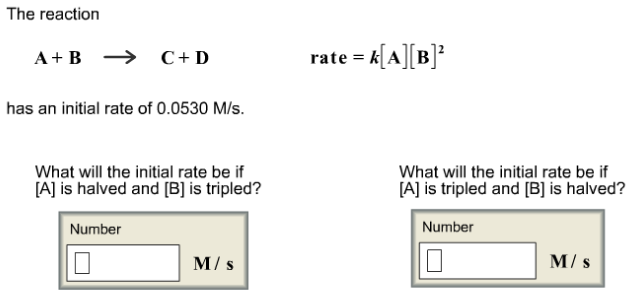# The reaction has an initial rate of 0.0530 M/s. What will the initial rate be if [A] is halved and [B] is tripled?

The reaction has an initial rate of 0.0530 M/s. What will the initial rate be if [A] is halved and [B] is tripled?Solution

Given data

Reaction equation

A+ B ----> C+D

Rate = k[A][B]2

Initial rate = 0.0530 M/s

What will the initial rate be if [A] is halved and [B] is tripled
What will the initial rate be if [A] is tripled and [B ] is halved

Lets first calculate the rate constant for the reaction

Assume concentration of the [A] and [B] = 1
Now lets use these concentration to calculate the initial rate constant

Rate = k[A][B]2
0.0530 M/s = k [ 1] 2
K = 0.0530 M/s / 1
K= 0.0530 M/s

Now lets use this rate constant and calculate the initial rates when the [A] is halved and [B] is tripled

So new concentration of [A] = 1 /2 = 0.5 M

And [B] = 1 * 3 = 3 M

Rate = k[A][B]2
Rate = 0.0530 M/s * [0.5] 2

Rate = 0.238 M/s

Now lets calculate rate when [A] is tripled and [B] is halved

Then concentration of the [A] = 3 m and [B] = 0.5 M
Lets use these concentrations and calculate the rate

Rate = k[A][B]2
Rate = 0.0530 M/s*[0.5]2

Rate = 0.0398 M/s